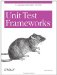# 8.4 Test Assert Methods

### 8.4 Test Assert Methods

NUnit provides a variety of test assert methods. Each one has a variant taking a message parameter, which appears as a descriptive message when the test fails.

The test assert methods referenced here are static methods of the class Assert . Additional test assert methods exist in the class Assertion , but they are obsolete and not recommended for use.

IsTrue(bool condition)

IsTrue(bool condition, string message)

Test passes if condition is true . This is the most generic type of assert.

IsFalse(bool condition)

IsFalse(bool condition, string message)

Test passes if condition is false .

AreEqual(decimal expected, decimal actual)

AreEqual(decimal expected, decimal actual, string message)

AreEqual(int expected, int actual)

AreEqual(int expected, int actual, string message)

Test passes if expected and actual are numerically equal.

AreEqual(double expected, double actual, double delta)

AreEqual(double expected, double actual, double delta, string message)

AreEqual(float expected, float actual, float delta)

AreEqual(float expected, float actual, float delta, string message)

Test passes if expected and actual are numerically equal within a tolerance of delta . If delta is 0, exact equality is necessary for test to pass.

AreEqual(Object expected, Object actual)

AreEqual(Object expected, Object actual, string message)

Test passes if expected and actual are equal. If both are numeric types, they are tested for numerical equality. Otherwise, the method Object.equals( ) is used to test equality.

AreSame(Object expected, Object actual)

AreSame(Object expected, Object actual, string message)

Test passes if expected and actual refer to the same Object .

IsNotNull(Object anObject)

IsNotNull(Object anObject, string message)

Test passes if anObject is not null .

IsNull(Object anObject)

IsNull(Object anObject, string message)

Test passes if anObject is null .

Fail( )

Fail(string message)

Test assert that always fails.Unit Test Frameworks
ISBN: 0596006896
EAN: 2147483647
Year: 2006
Pages: 146
Authors: Paul Hamill